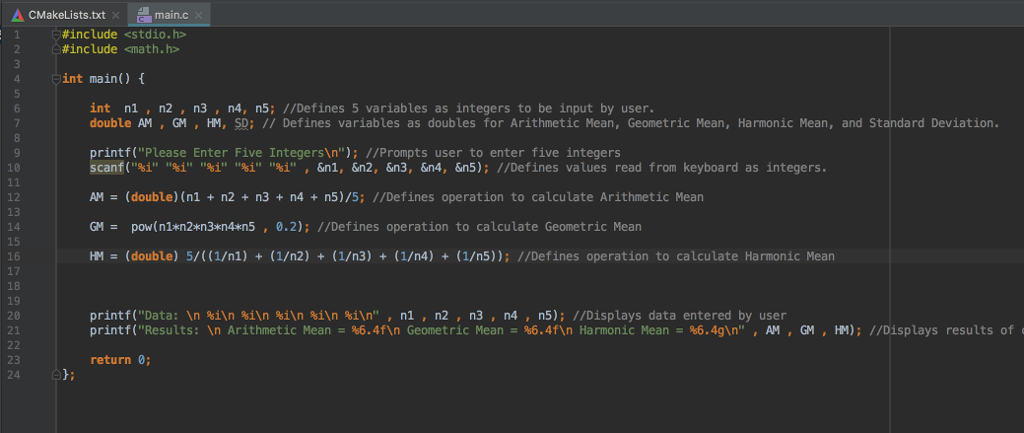# I am trying to create a program in C that calculates the Arithmetic Mean, Geometric Mean, Harmonic MI am trying to create a program in C that calculates the Arithmetic Mean, Geometric Mean, Harmonic Mean, and Standard Deviation (code not shown in picture). After running the program and entering the five integers, the program returns Geometric Mean = nan and Harmonic Mean = inf. I believe the issue has to do with the fact that the multiplication in the operations creates too large of integers for the program to store, but I’m not sure how to correct this for the program to run properly.

Also, when I input the operation for standard deviation, it says that the (^) binary operator cannot be used in double, but I need the double format to be able to get a floating point result since the input values need to be integers.

x A 2 CMakeLists.txt main.c #include #include math.h> oint main() { int n1 , n2 , n3 , n4, n5 ; M/Defines 5 variables as integers to be input by user, double AM , GM, HM, SD; TI Defines variables as doubles for Arithmetic Mean , Geometric Mean, Harmonic Mean , and Standard Deviation rintf(“Please Enter Five Integersn “): I/Prompts user to enter five integers scantf(“21” “1” “21” “i” “i”, Sni, An2, &n3, An4, &n5 ): I/Defines values read from keyboard as integers. AM (double) (ni + n2+ n3+ n4+ n5)/5; I/Defines operation to calculate Arithmetic Means GM = pow(nlan2n3 nzen4%n5 , 0.2): I/Defines operation to calculate Geometric Mean HMS (double) 5/((1/n2) + (1/n2) + (1/n3) + (1/n4) + (1/n5): T/Defines operation to calculate Harmonic Mean printf (‘Data: In hin in in in in “, nin2, n3 , no, n5 ): I/Displays data entered by user printf (“Results: In Arithmetic Mean B 6.4fn Geometric Mean B 6.4n Harmonic Mean = 6.4gn “, AM, GM, HM): ;1/Displays results of return 0;

I have coded your program as per your expectation. Let me know if you have any concern: –

#include<stdio.h>

#include<math.h>

int main() {

double a,b,c,d,e; // Define 5 variables

double am,gm,hm,sd,mean; // define Arithematic mean, Geometric mean, Harmonic Mean, Standard Deviation, Mean

printf(“Enter five numbers: n”);

scanf(“%lf”,&a);

scanf(“%lf”,&b);

scanf(“%lf”,&c);

scanf(“%lf”,&d);

scanf(“%lf”,&e);

int a1,b1,c1,d1,e1;

a1 = (int)a;

b1 = (int)b;

c1 = (int)c;

d1 = (int)d;

e1 = (int)e;

printf(“nnData: %d %d %d %d %dn”,a1,b1,c1,d1,e1);

am = (a+b+c+d+e)/ 5.0;

gm = pow((a*b*c*d*e),(1.0/5.0));

hm = 5.0/(1.0/a+1.0/b+1.0/c+1.0/d+1.0/e);

mean = (a + b + c + d + e) / 5.0;

sd = sqrt(((pow(a-mean,2)) + (pow(b-mean,2)) + (pow(c-mean,2)) + (pow(d-mean,2)) + (pow(e-mean,2)))/ 5.0);

printf(“Results: n Arithmatic Mean = %6.4fn Geometric Mean = %6.4fn Harmonic Mean = %6.4fn Standard Deviation = %6.4fn”,am,gm,hm,sd);

return 0;

}

Sample Output

Enter five numbers:
85
43
95
100
78

Data: 85 43 95 100 78
Results:
Arithmatic Mean = 80.2000
Geometric Mean = 77.0089
Harmonic Mean = 73.1343
Standard Deviation = 20.1137Pages (550 words)
Approximate price: -

Help Me Write My Essay - Reasons:Best Online Essay Writing Service

We strive to give our customers the best online essay writing experience. We Make sure essays are submitted on time and all the instructions are followed.Our Writers are Experienced and Professional

Our essay writing service is founded on professional writers who are on stand by to help you any time.Free Revision Fo all Essays

Sometimes you may require our writers to add on a point to make your essay as customised as possible, we will give you unlimited times to do this. And we will do it for free.Timely Essay(s)

We understand the frustrations that comes with late essays and our writers are extra careful to not violate this term. Our support team is always engauging our writers to help you have your essay ahead of time.Customised Essays &100% Confidential

Our Online writing Service has zero torelance for plagiarised papers. We have plagiarism checking tool that generate plagiarism reports just to make sure you are satisfied.Try it now!

## Calculate the price of your order

Total price:
\$0.00

How it works?Fill in the order form and provide all details of your assignment.Proceed with the payment

Choose the payment system that suits you most.HOW OUR ONLINE ESSAY WRITING SERVICE WORKS

Let us write that nagging essay.By clicking on the "PLACE ORDER" button, tell us your requires. Be precise for an accurate customised essay. You may also upload any reading materials where applicable.Pick A & Writer

Our ordering form will provide you with a list of writers and their feedbacks. At step 2, its time select a writer. Our online agents are on stand by to help you just in case.Editing (OUR PART)

At this stage, our editor will go through your essay and make sure your writer did meet all the instructions.